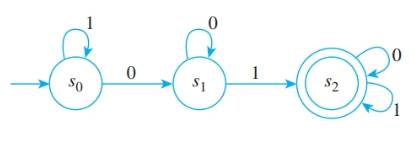Chapter 12.2, Problem 2ES### Discrete Mathematics With Applicat...

5th Edition
EPP + 1 other
ISBN: 9781337694193

#### Solutions

Chapter
Section### Discrete Mathematics With Applicat...

5th Edition
EPP + 1 other
ISBN: 9781337694193
Textbook Problem
1 views

# In 2—7, a finite-state automaton is given by a transition diagram. For each automaton: a. Find its states. b. Find its input symbols. C. Find its initial state. d. Find its accepting states. e. Write its annotated next-state table. 2.To determine

(a)

To find:

The states of given finite-state automation.

Explanation

Given information:

A finite-state automation is given by a transition diagram as shown below:

Calculation:

The states are written in circle...

To determine

(b)

To find:

The input symbols of given finite-state automation.

To determine

(c)

To find:

The initial states of given finite-state automation.

To determine

(d)

To find:

The accepting states of given finite-state automation.

To determine

(e)

To write:

The annotated next-state table of given finite-state automation.

### Still sussing out bartleby?

Check out a sample textbook solution.

See a sample solution

#### The Solution to Your Study Problems

Bartleby provides explanations to thousands of textbook problems written by our experts, many with advanced degrees!

Get Started